Courses

# Homogeneous and Heterogeneous Catalysis - Chemical Kinetics Chemistry Notes | EduRev

## Chemistry : Homogeneous and Heterogeneous Catalysis - Chemical Kinetics Chemistry Notes | EduRev

The document Homogeneous and Heterogeneous Catalysis - Chemical Kinetics Chemistry Notes | EduRev is a part of the Chemistry Course Physical Chemistry.
All you need of Chemistry at this link: Chemistry

Homogeneous and Heterogeneous Catalysis.

A homogeneous catalyst is a catalyst that exist in the same phase as the species involved in the reaction, and heterogeneous catalysts exist in a different phase. Enzymes surve as an example of a homogeneous catalyst, they exist in solution and catalyze reactions that occur in solution.
In heterogeneous catalysis reaction, an important step in reactions involving solid catalysis is the absorption of one or more of the reactants to the solid surface. The particles absorb to the surface without changing their internal bonding. An equilibrium exists between the free and surfaceabsorbed species or adsorbate and surface adsorption and deadsorption can be obtained.
A critical parameter in evaluating surface adsorption is the fractional coverage, θ, defined as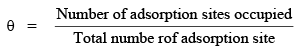The variation of θ with pressure at fixed temperature is called adsorption isotherm.

(a)  The Langmuir Isotherm : The simplest kinetic model describing the adsorption process is known as the Langmuir model, where adsorption is described by the following mechanism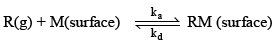R is reagent, M is an unoccupied absorption site of catalyst and RM is an occupied adsorption site. ka and kd is the rate constant for adsorption and deadsorption.
Three approximations are employed in the Langmuir model:

(1)  Adsorption is complete once monolayer coverage has been reached.

(2)  All adsorption site are equivalent and the surface is uniform

The rate of change in θ will depends on the rate constant for adsorption ka, reagent pressure P and the number of vacant site which is equal to the total number of adsorption sites, N, times the fraction of sites that are open (1 – θ)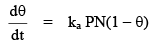The change in θ due to deadsorption is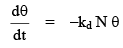At equilibrium, the change in θ with time is zero i.e

. (ka PN + kdN) θ = kaPN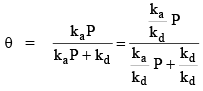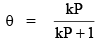where k is the equilibrium constant defined as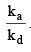This equation is the equation for the Langmuir isotherm.
In many instances adsorption is accompanied by dissociation of the adsorbate, a process that is described by the following mechanism: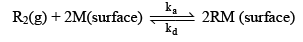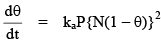&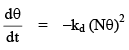The condition for no net change leads to the isotherm.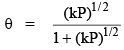i.e. the surface coverage now depends more weakly on pressure than for non-dissociative adsorption.

(b)  The BET isotherm.
If initial adsorbed layer can be act as a substance for further  adsorption, then, instead of the isotherm leveling off the some saturated value at high pressure, it can be expected to rise

indefinitely. The most widely used isotherm dealing with multiplayer adsorption was derived by Brunauer, Emmett and Teller, and is called the BET isotherm.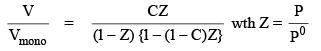P° = vapour pressure above a layer of absorbate
Vmono = volume of monolayer coverage.
C = constant The Langmuir-Hinshelwood mechanism for adsorption and catalysis.

(1)  Unimolecular surface Reaction.
A is reactant and S is the vacant site on surface.
If r is the rate of the reaction, then according to the Langnuir-H. hypothesis,

r ∝ θ

r = k2θ

Apply S.S.A. for the formation of [AS].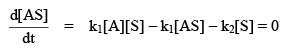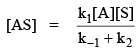If Cs is the total concentration of act ive site on surface, then the concentration [S] of the vacant sites on the surface is equal to the product of Cs and (1 – θ). Thus

[S] = Cs (1 - θ)

Also, the concentration of AS on the surface is,

[AS] = Csθ

then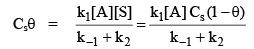or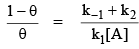or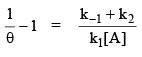or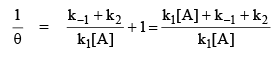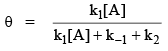…(1)
thus,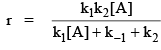...(2)

The concentration is expressed in terms of partial pressure
Then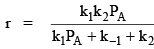or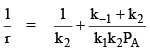...(3)

Two limiting cases

Case I.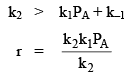∴         r = k1PA it is first order w.r.t. A

Case II. k2 << k1P+ k–1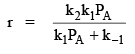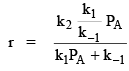∵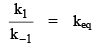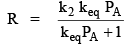Two situations arise depending upon the pressure:

(1)  At low pressure θ → 0 and keq PA << 1 so that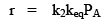It is first order w.r.t. PA or [A].

(a) At high pressure; θ → 1 and keqP>> 1 so that

r = k2
it is zero order with respect to PA or [A].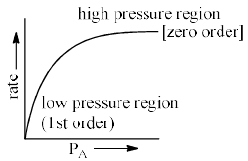(2)  Bimolecular surface reaction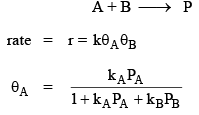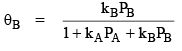then it follow the rate law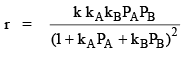Offer running on EduRev: Apply code STAYHOME200 to get INR 200 off on our premium plan EduRev Infinity!

## Physical Chemistry

66 videos|94 docs|32 tests

,

,

,

,

,

,

,

,

,

,

,

,

,

,

,

,

,

,

,

,

,

;